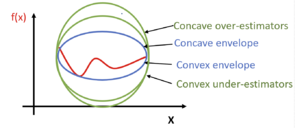# McCormick envelopes

Author: Susan Urban (SYSEN 5800 Fall 2021)

## Introduction

The McCormick Envelope, originally developed by Dr. Garth McCormick, is a type of convex relaxation used for the optimization of bilinear $(e.g.,x*y,e^{x}y+y,sin(x+y)-x^{2})$non-linear programming (NLP) problems1. Optimization of these non-convex functions f(x) is challenging since they may have multiple locally optimal solutions or no solution. It can take a significant amount of time, computing resources, and effort to determine if the solution is global or the problem has no feasible solution2. Different techniques are used to address this challenge depending on the characteristics of the problem.

## Theory, Methodology and Algorithmic DiscussionsFigure 1: Relationships between the given function, concave over-estimators, convex under-estimators, a concave envelope, and a convex envelope.

As McCormick noted, McCormick Envelopes are based on the key assumption that convex and concave envelopes can be constructed for the given function1. The concave envelope, and respectively the convex envelope, is the concave over-estimator and convex under-estimator for the given function providing the tightest fit to the given function1. The envelope surrounds the given function, like an envelope encloses a letter, and limits the feasible solution space the most in comparison to all other concave over-estimators and convex under-estimators. Multiple concave over-estimators and multiple convex under-estimators may exist but there is only one concave envelope and one convex envelope for a given function and domain. Figure 1 depicts the relationship between the given function f(x), multiple concave over-estimators, multiple convex under-estimators, a convex envelope, and a concave envelope.

Each bilinear term is replaced with a new variable and four sets of constraints are added1. The non-linear programming is converted to a relaxed convex linear programming (LP) which can be more easily solved.

The LP solution gives a lower (L) bound and any feasible solution to the problem gives an upper (U) bound.

As noted by Scott et al, McCormick envelopes are effective since they are recursive, can be applied to a variety of applications, and are typically stronger than those resulting from convexification or linearization procedures3.

The following is a derivation of the McCormick Envelopes for a given bilinear function1:

$Let\ w=xy$$x^{L}\leq x\leq x^{U}$$y^{L}\leq y\leq y^{U}$where $x^{L},x^{U},y^{L},y^{U}$are   upper  and   lower  bound  values  for  $x$and $y$, respectively.

$a=\left(x-x^{L}\right)$$b=\left(y-y^{L}\right)$a and b are both positive resulting in a positive product

$a*b\geq 0$$a*b=\left(x-x^{L}\right)\left(y-y^{L}\right)=xy-x^{L}y-xy^{L}+x^{L}y^{L}\geq 0$substituting w=xy and reformulating the inequality produces

$w\geq x^{L}y+xy^{L}-x^{L}y^{L}$Following the same sequence of steps, the remaining inequalities are produced:

$a=\left(x^{U}-x\right)$$b=\left(y^{U}-y\right)$$w\geq x^{U}y+xy^{U}-x^{U}y^{U}$$a=\left(x^{U}-x\right)$$b=\left(y-y^{L}\right)$$w\leq x^{U}y+xy^{L}-x^{U}y^{L}$$a=\left(x-x^{L}\right)$$b=\left(y^{U}-y\right)$$w\leq xy^{U}+x^{L}y-x^{L}y^{U}$The under-estimators of the function are represented by:

$w\geq x^{L}y+xy^{L}-x^{L}y^{L}$$w\geq x^{U}y+xy^{U}-x^{U}y^{U}$The over-estimators of the function are represented by:

$w\leq x^{U}y+xy^{L}-x^{U}y^{L}$$w\leq xy^{U}+x^{L}y-x^{L}y^{U}$The following shows the relaxation of a non-convex problem using McCormick Envelopes:

The original non-convex problem:

$\min Z=\textstyle \sum _{i=1}\sum _{j=1}c_{i,j}x_{i}x_{j}+g_{0}(x)$$s.t.\sum _{i=1}\sum _{j=1}c_{i,j}^{l}x_{i}x_{j}+g_{l}(x)\leq 0,\forall l\in L$$x^{L}\leq x\leq x^{U}$Replacing $u_{i,j}=x_{i}x_{j}$, we obtain a relaxed, convex problem using McCormick Envelopes:

$\min Z=\textstyle \sum _{i=1}\sum _{j=1}c_{i,j}u_{i,j}+g_{0}(x)$$s.t.\sum _{i=1}\sum _{j=1}c_{i,j}^{l}u_{i,j}+g_{l}(x)\leq 0,\forall l\in L$$u_{i,j}\geq x_{i}^{L}x_{j}+x_{i}x_{j}^{L}-{x_{i}}^{L}{x_{j}}^{L},\forall i,j\$$u_{i,j}\geq x_{i}^{U}x_{j}+x_{i}x_{j}^{U}-{x_{i}}^{U}{x_{j}}^{U},\forall i,j\$$u_{i,j}\leq x_{i}^{L}x_{j}+x_{i}x_{j}^{U}-{x_{i}}^{L}{x_{j}}^{U},\forall i,j\$$u_{i,j}\leq x_{i}^{U}x_{j}+x_{i}x_{j}^{L}-{x_{i}}^{U}{x_{j}}^{L},\forall i,j\$$x^{L}\leq x\leq x^{U},\ \ u^{L}\leq u\leq u^{U}$Similar steps are taken to derive the McCormick Envelopes for given functions with different formats e.g., exy.

Good lower and upper bounds focus and minimize the feasible solution space; they reduce the number of iterations to find the optimal solution.

Piecewise McCormick Relaxation

As discussed by Hazaji, global optimization solvers focus initially on optimizing the lower and upper bounds, and when necessary, focus on domain partitioning4. By dividing the domain of a given variable into partitions or smaller regions, the solver is able to tailor and further tighten the convex relaxations of each partition. This strategy is known as the Piecewise McCormick relaxation5.

## Example: Numerical

For the given problem,

$min\ Z=xy+6x+y$$s.t.\ xy\leq 18$$0\leq x\leq 10$$0\leq y\leq 2$$Let\ w=xy$$x^{L}=0$$x^{U}=10$$y^{L}=0$$y^{U}=2$Substituting these values into the original problem and the McCormick Envelopes, the problem is reformulated:

$min\ Z=w+6x+y$$s.t.\ w\leq 18$$w\geq x^{L}y+xy^{L}-x^{L}y^{L}$$w\geq 0*y+x*0-0*0$$w\geq 0$$w\geq x^{U}y+xy^{U}-x^{U}y^{U}$$w\geq 10*y+x*2-10*2$$w\geq 10y+2x-20$$w\leq x^{U}y+xy^{L}-x^{U}y^{L}$$w\leq 10*y+x*0-10*0$$w\leq 10y$$w\leq xy^{U}+x^{L}y-x^{L}y^{U}$$w\leq x*2+0*y-0*2$$w\leq 2x$Using GAMS to solve the reformulated problem, the solution is z= -76.2, x=10, y=1.8.

## Applications

Bilinear functions occur in numerous engineering and natural science applications where McCormick Envelopes can be utilized.

For example, an application involves the modeling of dynamic biological processes based on experimental data2. The challenge for this model is establishing model parameter values that align or calibrate the model response with observed data. These model parameter values can be estimated using optimization techniques, including McCormick Envelopes, to minimize the difference between observed and simulated data.

Another application involves the optimization of financial objectives and risk management for energy conversion for a large scale operation such as a college campus or a municipality6. This operation involves flexibility of energy sources and several facets of uncertainty involved with pricing. This model uses McCormick Envelopes to optimize the bilinear nonconvex components.

A third application involves the optimized efficiency and reliability of the operation of the electricity grid. Optimal power flow (OPF) and unit commitment (UC) are two optimization problems that support the electricity market operations7. McCormick Envelopes are used to strengthen the second-order cone (SOC) relaxation of the alternate current optimal power flow (ACOPF)8.

## Conclusion

Non-convex NLPs are challenging to solve and may require a significant amount of time, computing resources, and effort to determine if the solution is global or the problem has no feasible solution. McCormick Envelopes provide a relaxation technique for bilinear non-convex nonlinear programming problems. McCormick Envelopes provide a straightforward technique of replacing each bilinear term with a new variable and adding four constraints. Due to the recursive nature of this technique, it may be applied to a wide variety of engineering and scientific applications involving bilinear terms.